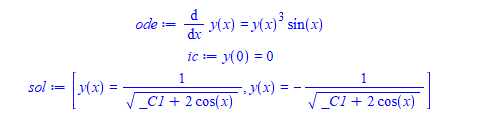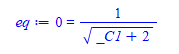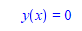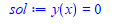# Question:Can one obtain infinity as a solution for a variable in an equation?

## Question:Can one obtain infinity as a solution for a variable in an equation?

Maple 2021

sometimes I get a solution to an ODE, where when I plugin the initial conditions to solve for the constant of integration, the solution by inspection can be seen to be infinity.

The constant of integration is allowed to be infinity.

But now I get no solution from solve and so my program rejects this solution and says the ode can't be solved because it can't find value for the constant of integration using this initial conditions.

I am not sure how to handle this, since Maple solve will not return an infinity as possible solution for the constant of integration. I tried different assumptions.

This has to work for general, and not for this specific example I will show now.

Here is an ODE with IC

```ode:=diff(y(x),x)=y(x)^3*sin(x);
ic:=y(0)=0;
sol:=[dsolve(ode)];```The general solution is easy to find since this is just separable. The problem is when I plugin the initial conditions. (again, this is done in a program, without looking at the screen). let consider the first solution above for now.

`eq:=simplify(subs( [y(x)=0,x=0],sol));`And here is the problem, when next I try to solve for the constant, Maple says no solution.

`solve(eq,_C1,allsolutions=true) assuming _C1::real`

No solution returned.

If Maple could return _C1=infinity, then I would plugin _C1=infinity and obtain the correct particular solution which is

`simplify(subs(_C1=infinity,sol)) assuming real`Which is what the smart dsolve command does

```ode:=diff(y(x),x)=y(x)^3*sin(x);
ic:=y(0)=0;
sol:=dsolve([ode,ic])```I do not know how Maple found the above and what algorithm it used.

But my not too smart dsolve program gets stuck on such problems, because it can't solve for the IC.

Is there another method or option or assumptions to use, to solve such an equation and request infinity be returned as a solution?  I tried isolate(eq,_C1) but that did not work.

One possible option to try as a last resource is to do this

`limit(eq,_C1=infinity)`

And this gives 0=0

which means _C1=infinity is valid solution.  And I could do this as final attempt before giving up. Is this what Maple did? But how does one know to do this in general? Unless one tries and find out? i.e I could try limit(eq,_C1=infinity) and limit(eq,_C1=-infinity) and see if any one of these gives true (after doing  evalb or is  on the output)

Any suggestions or a better/more robust method to use for such cases? I need to use the method for any equation where infinity can be a solution for the constant of integration. It will always be one equation and one variable, and can assume all are real for now if it makes it easier.﻿